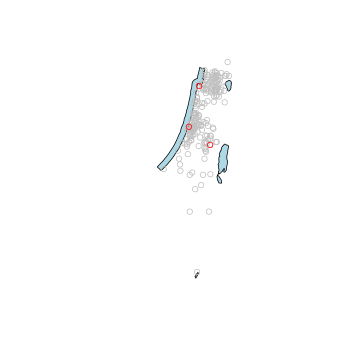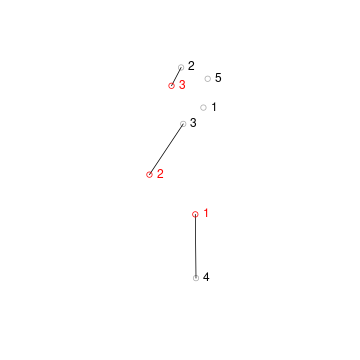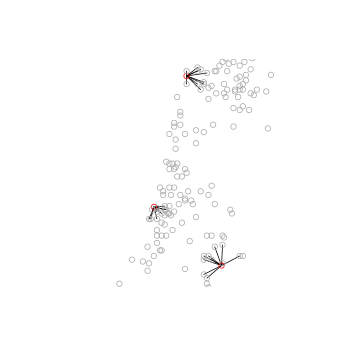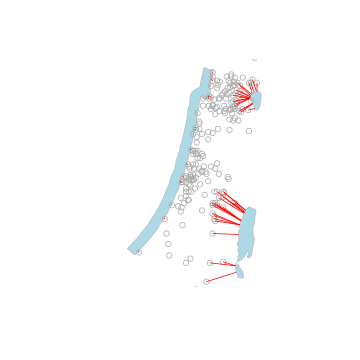# Introduction

## Package purpose

This document introduces the nngeo package. The nngeo package includes functions for spatial join of layers based on k-nearest neighbor relation between features. The functions work with spatial layer object defined in package sf, namely classes sfc and sf.

## Installation

CRAN version:

install.packages("remotes")
remotes::install_github("michaeldorman/nngeo")


GitHub version:

install.packages("nngeo")


## Sample data

The nngeo package comes with three sample datasets:

• cities
• towns
• water

The cities layer is a point layer representing the location of the three largest cities in Israel.

cities
#> Simple feature collection with 3 features and 1 field
#> geometry type:  POINT
#> dimension:      XY
#> bbox:           xmin: 34.78177 ymin: 31.76832 xmax: 35.21371 ymax: 32.79405
#> geographic CRS: WGS 84
#>        name                  geometry
#> 1 Jerusalem POINT (35.21371 31.76832)
#> 2  Tel-Aviv  POINT (34.78177 32.0853)
#> 3     Haifa POINT (34.98957 32.79405)


The towns layer is another point layer, with the location of all large towns in Israel, compiled from a different data source:

towns
#> Simple feature collection with 193 features and 4 fields
#> geometry type:  POINT
#> dimension:      XY
#> bbox:           xmin: 34.27 ymin: 29.56 xmax: 35.6 ymax: 33.21
#> geographic CRS: WGS 84
#> First 10 features:
#>             name country.etc   pop capital            geometry
#> 12        'Afula      Israel 39151       0 POINT (35.29 32.62)
#> 17         'Akko      Israel 45606       0 POINT (35.08 32.94)
#> 40       'Ar'ara      Israel 15841       0  POINT (35.1 32.49)
#> 41         'Arad      Israel 22757       0 POINT (35.22 31.26)
#> 43       'Arrabe      Israel 20316       0 POINT (35.33 32.85)
#> 52        'Atlit      Israel  4686       0 POINT (34.93 32.68)
#> 103     'Eilabun      Israel  4296       0  POINT (35.4 32.83)
#> 104   'Ein Mahel      Israel 11014       0 POINT (35.35 32.72)
#> 105 'Ein Qiniyye      Israel  2101       0 POINT (35.15 31.93)
#> 112        'Ilut      Israel  6536       0 POINT (35.25 32.72)


The water layer is an example of a polygonal layer. This layer contains four polygons of water bodies in Israel.

water
#> Simple feature collection with 4 features and 1 field
#> geometry type:  POLYGON
#> dimension:      XY
#> bbox:           xmin: 34.1388 ymin: 29.45338 xmax: 35.64979 ymax: 33.1164
#> geographic CRS: WGS 84
#>                name                       geometry
#> 1           Red Sea POLYGON ((34.96428 29.54775...
#> 2 Mediterranean Sea POLYGON ((35.10533 33.07661...
#> 3          Dead Sea POLYGON ((35.54743 31.37881...
#> 4    Sea of Galilee POLYGON ((35.6014 32.89248,...


Figure \ref{fig:layers} shows the spatial configuration of the cities, towns and water layers.

plot(st_geometry(water), col = "lightblue")
plot(st_geometry(towns), col = "grey", pch = 1, add = TRUE)
plot(st_geometry(cities), col = "red", pch = 1, add = TRUE)# Usage examples

## The st_nn function

The main function in the nngeo package is st_nn.

The st_nn function accepts two layers, x and y, and returns a list with the same number of elements as x features. Each list element i is an integer vector with all indices j for which x[i] and y[j] are nearest neighbors.

For example, the following expression finds which feature in towns[1:5, ] is the nearest neighbor to each feature in cities:

nn = st_nn(cities, towns[1:5, ], progress = FALSE)
#> lon-lat points
nn
#> []
#>  4
#>
#> []
#>  3
#>
#> []
#>  2


This output tells us that towns[4, ] is the nearest among the five features of towns[1:5, ] to cities[1, ], etc.

## The st_connect function

The resulting nearest neighbor matches can be visualized using the st_connect function. This function builds a line layer connecting features from two layers x and y based on the relations defined in a list such the one returned by st_nn:

l = st_connect(cities, towns[1:5, ], ids = nn)
#> Calculating nearest IDs
#> Calculating lines
l
#> Geometry set for 3 features
#> geometry type:  LINESTRING
#> dimension:      XY
#> bbox:           xmin: 34.78177 ymin: 31.26 xmax: 35.22 ymax: 32.94
#> geographic CRS: WGS 84
#> LINESTRING (35.21371 31.76832, 35.22 31.26)
#> LINESTRING (34.78177 32.0853, 35.1 32.49)
#> LINESTRING (34.98957 32.79405, 35.08 32.94)


Plotting the line layer l gives a visual demonstration of the nearest neighbors match, as shown in Figure \ref{fig:st_connect}.

plot(st_geometry(towns[1:5, ]), col = "darkgrey")
plot(st_geometry(cities), col = "red", add = TRUE)
text(st_coordinates(cities)[, 1], st_coordinates(cities)[, 2], 1:3, col = "red", pos = 4)
text(st_coordinates(towns[1:5, ])[, 1], st_coordinates(towns[1:5, ])[, 2], 1:5, pos = 4)## Dense matrix representation

The st_nn can also return the complete logical matrix indicating whether each feature in x is a neighbor of y. To get the dense matrix, instead of a list, use sparse=FALSE.

nn = st_nn(cities, towns[1:5, ], sparse = FALSE, progress = FALSE)
#> lon-lat points
nn
#>       [,1]  [,2]  [,3]  [,4]  [,5]
#> [1,] FALSE FALSE FALSE  TRUE FALSE
#> [2,] FALSE FALSE  TRUE FALSE FALSE
#> [3,] FALSE  TRUE FALSE FALSE FALSE


## k-Nearest neighbors where k>0

It is also possible to return any k-nearest neighbors, rather than just one. For example, setting k=2 returns both the 1st^ and 2nd^ nearest neighbors:

nn = st_nn(cities, towns[1:5, ], k = 2, progress = FALSE)
#> lon-lat points
nn
#> []
#>  4 3
#>
#> []
#>  3 1
#>
#> []
#>  2 5

nn = st_nn(cities, towns[1:5, ], sparse = FALSE, k = 2, progress = FALSE)
#> lon-lat points
nn
#>       [,1]  [,2]  [,3]  [,4]  [,5]
#> [1,] FALSE FALSE  TRUE  TRUE FALSE
#> [2,]  TRUE FALSE  TRUE FALSE FALSE
#> [3,] FALSE  TRUE FALSE FALSE  TRUE


## Distance matrix

Using returnDist=TRUE the distances list is also returned, in addition the the neighbor matches, with both components now comprising a list:

nn = st_nn(cities, towns[1:5, ], k = 2, returnDist = TRUE, progress = FALSE)
#> lon-lat points
nn
#> $nn #>$nn[]
#>  4 3
#>
#> $nn[] #>  3 1 #> #>$nn[]
#>  2 5
#>
#>
#> $dist #>$dist[]
#>  56364.74 80742.62
#>
#> $dist[] #>  53968.63 76186.87 #> #>$dist[]
#>  18265.72 32476.24


Finally, the search for nearest neighbors can be limited to a search radius using maxdist. In the following example, the search radius is set to 50,000 meters (50 kilometers). Note that no neighbors are found within the search radius for cities[2, ]:

nn = st_nn(cities, towns[1:5, ], k = 2, maxdist = 50000, progress = FALSE)
#> lon-lat points
nn
#> []
#> integer(0)
#>
#> []
#> integer(0)
#>
#> []
#>  2 5


## Spatial join

The st_nn function can also be used as a geometry predicate function when performing spatial join with sf::st_join. For example, the following expression spatially joins the two nearest towns[1:5, ] features to each cities features, using a search radius of 50 km:

cities1 = st_join(cities, towns[1:5, ], join = st_nn, k = 2, maxdist = 50000)
#> lon-lat points


Here is the resulting layer:

cities1
#> Simple feature collection with 4 features and 5 fields
#> geometry type:  POINT
#> dimension:      XY
#> bbox:           xmin: 34.78177 ymin: 31.76832 xmax: 35.21371 ymax: 32.79405
#> geographic CRS: WGS 84
#>        name.x  name.y country.etc   pop capital                  geometry
#> 1   Jerusalem    <NA>        <NA>    NA      NA POINT (35.21371 31.76832)
#> 2    Tel-Aviv    <NA>        <NA>    NA      NA  POINT (34.78177 32.0853)
#> 3       Haifa   'Akko      Israel 45606       0 POINT (34.98957 32.79405)
#> 3.1     Haifa 'Arrabe      Israel 20316       0 POINT (34.98957 32.79405)


## Another example

Here is another example, finding the 10-nearest neighbor towns features for each cities feature:

x = st_nn(cities, towns, k = 10)
#> lon-lat points
l = st_connect(cities, towns, ids = x)


The result is visualized in Figure \ref{fig:cities_towns}.

plot(st_geometry(l))
plot(st_geometry(cities), col = "red", add = TRUE)
plot(st_geometry(towns), col = "darkgrey", add = TRUE)## Polygons

Nearest neighbor search also works for non-point layers. The following code section finds the 20-nearest towns features for each water body in water[-1, ].

nn = st_nn(water[-1, ], towns, k = 20, progress = FALSE)
#> lines or polygons


Again, we can calculate the respective lines for the above result using st_connect. Since one of the inputs is line/polygon, we need to specify a sampling distance dist, which sets the resolution of connecting points on the shape exterior boundary.

l = st_connect(water[-1, ], towns, ids = nn, dist = 100)
#> Calculating nearest IDs
#> Calculating lines


The result is visualized in Figure \ref{fig:water_towns}.

plot(st_geometry(water[-1, ]), col = "lightblue", border = "grey")
plot(st_geometry(towns), col = "darkgrey", add = TRUE)
plot(st_geometry(l), col = "red", add = TRUE)